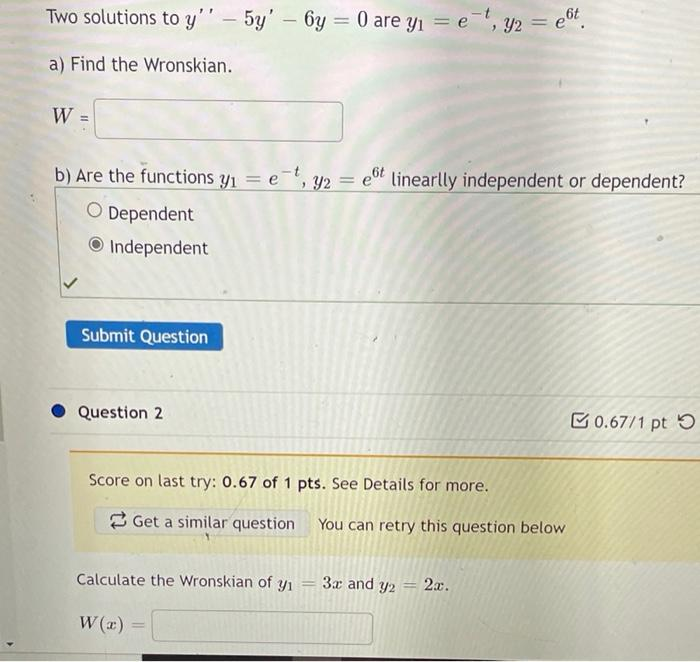# Question both Two solutions to y'' – 5y' – 6y = 0 are yı = e-, y2 = e(t. a) Find the Wronskian. W b) Are the functions yı = e-t, y2 = et linearlly independent or dependent? ? O Dependent Independent Submit Question Question 2 0.67/1 pt Score on last try: 0.67 of 1 pts. See Details for more. Get a similar question You can retry this question below Calculate the Wronskian of yı 3.c and y2 2a. W(x)